Example Questions

Example Question #21 : Algebra

What digit appears in the units place when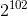is multiplied out?Explanation:

This problem is quite simple if you recall that the units place of powers of 2 follows a simple 4-step sequence.

Observe the first few powers of 2:

2= 2, 2= 4, 2= 8, 2= 16, 2= 32, 2= 64, 2= 128, 2= 256 . . .

The units place follows a sequence of 2, 4, 8, 6, 2, 4, 8, 6, etc. Thus, divide 102 by 4. This gives a remainder of 2.

The second number in the sequence is 4, so the answer is 4.

Example Question #21 : Algebra

Which of the following is a multiple of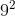?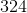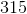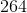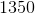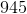Explanation:

For exponent problems like this, the easiest thing to do is to break down all the numbers that you have into their prime factors. Begin with the number given to you: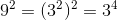Now, in order for you to have a number that is a multiple of this, you will need to have at least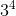in the prime factorization of the given number.  For each of the answer choices, you have: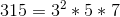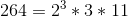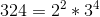; This is the answer.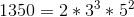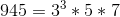Example Question #1 : How To Find Patterns In Exponents

Simplify the following: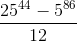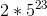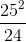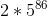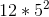Explanation:

Because the numbers involved in your fraction are so large, you are going to need to do some careful manipulating to get your answer. (A basic calculator will not work for something like this.) These sorts of questions almost always work well when you isolate the large factors and notice patterns involved. Let's first focus on the numerator. Go ahead and break apart theinto its prime factors: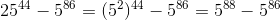Note that these have a common factor of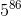. Therefore, you can rewrite the numerator as: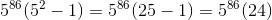Now, put this back into your fraction: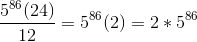Example Question #21 : Gre Quantitative Reasoning

Quantitative Comparison

Quantity A: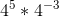Quantity B: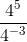The relationship cannot be determined from the information given.

Quantity A is greater.

Quantity B is greater.

The two quantities are equal.

Quantity B is greater.

Explanation:

In Quantity A, we add the exponents because the operation is multiplication, so 45 * 4–3 = 45+(–3) = 42. In Quantity B, we subtract the exponents because the operation is division, so 45 / 4= 45–(–3) = 48. We don't have to finish multiplying out the exponents to see that Quantity B is greater.

Example Question #2 : How To Find A Ratio Of Exponents

If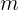andare both rational numbers and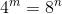, what is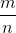?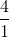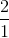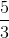Explanation:

This question is asking you for the ratio of m to n.  To figure it out, the easiest way is to figure out when 4 to an exponent equals 8 to an exponent.  The easiest way to do that is to list the first few results of 4 to an exponent and 8 to an exponent and check to see if any match up, before resorting to more drastic means of finding a formula.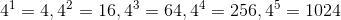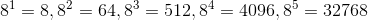And, would you look at that.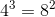.  Therefore,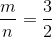.

Example Question #1 : Exponents And Rational Numbers

find x

8x=2x+6

3

2

-1

2 or -1

4

3

Explanation:

8 = 23

(23)x = 23x

23x = 2x+6  <- when the bases are the same, you can set the exponents equal to each other and solve for x

3x=x+6

2x=6

x=3

Example Question #2 : Exponents And Rational Numbers

Compare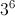and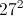.

The relationship cannot be determined from the information given.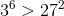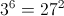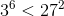Explanation:

First rewrite the two expressions so that they have the same base, and then compare their exponents.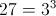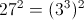Combine exponents by multiplying: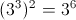This is the same as the first given expression, so the two expressions are equal.

Example Question #3 : Exponents And Rational Numbers

Solve for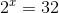Explanation:can be written as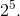Since there is a common base of, we can say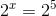orExample Question #21 : Algebra

Solve for.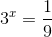Explanation:

The basees don't match.

However: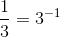thus we can rewrite the expression as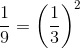.

Anything raised to negative power meansover the base raised to the postive exponent.

So,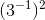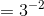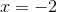Example Question #5 : Exponents And Rational Numbers

Solve for.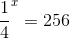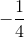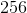Explanation:

The bases don't match.

However: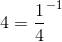and we recognize that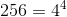.

Anything raised to negative power meansover the base raised to the postive exponent.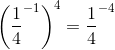.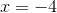Tired of practice problems?

Try live online GRE prep today.